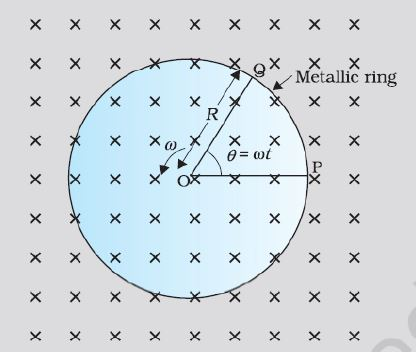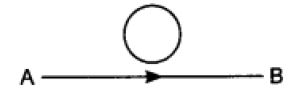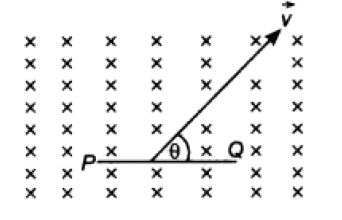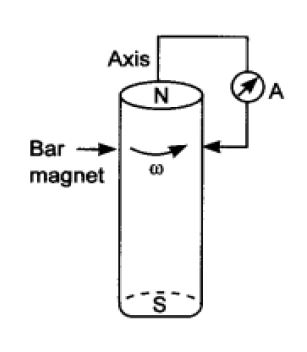# MCQ Electro Magnetic Induction Class 12 Physics Chapter 6

### MCQ Electro Magnetic Induction Class 12 Physics Chapter 6

1. Lenz’s law of electromagnetic induction is as per law of conservation of

a) energy

b) angular momentum

c) charge

d) electromotive force.

Ans. (a) energy

Explanation: Against to the opposing force work is done. The work done by the external agency get converted in to Electrical energy.

2. Which of the following statements is not correct?

a) Whenever the amount of magnetic flux linked with a circuit changes, an emf is induced in circuit.

b) The induced emf lasts so long as the change in magnetic flux continues.

c) The direction of induced emf is given by Lenz’s law.

d) Lenz’s law is a consequence of the law of conservation of momentum.

Ans. (d) Lenz’s law is a consequence of the law of conservation of momentum.

Explanation: Conservation of Angular momentum ( I x ω = constant) has nothing to do with Lenz’s law

3. Which of the following does not use the application of eddy current?

a) Electric power meters

b) Induction furnace

c) LED lights

d) Magnetic brakes in trains

Ans.  (c) LED lights

Explanation : Eddy current cannot make an LED glow.

4. A metallic rod of length ‘ L’ is rotated with a angular frequency of ‘ ω ‘ , with one end hinged at the centre and the other end at the circumference of circular metallic ring of radius ‘R’, about an axis passing through the centre and perpendicular to the plane of the ring as shown in the figure. A constant and uniform magnetic field ‘B’ parallel to the axis is present everywhere. What is the emf between the centre and the metallic ring?a) BωR )/2

b) ( Bω2R )/2

c) ( BωR2)/2

d) ( B2ωR)/2

Ans. c) (BωR2)/2

Explanation: Simple derivation

5. In the given figure current from A to B in the straight wire is increasing. The direction of the induced current in the loop isa) clockwise

b) anticlockwise

c) straight line

d) no induced e.m.f. produced.

Ans: (a) clockwise.

Explanation: According to Lenz’s law

6. A rod PQ of length ‘L’ is moved in uniform magnetic field ‘B’ as shown.If the rod is moving with a velocity ‘v’ making an angle ‘θ’ with the magnetic field. What will be the emf induced in it?

a) BLvsinθ

b) BLvcosθ

c) BLv

d) BLvtanθ

Ans. a) BLvsinθ

Explanation: The component of velocity which contribute to the motional emf is v sinθ

7. When current in a coil changes from 5 A to 2 A in 0.1 s, averagevoltage of 50 V is produced. The self-inductance of the coil will be.

a) 1.67 H

b) 6 H

c) 3 H

d) 0.67 H

Ans. a) 1.67 H

Explanation:e = – L , By substituting the values of e, dI , dt the value of L can be calculated.

8. The north pole of a long bar magnet was pushed slowly into a short solenoid connected to a galvanometer. The magnet was heldstationary for a few seconds with the north pole in the middle of the solenoid and then withdrawn rapidly. The maximum deflection of the galvanometer was observed when the magnet was

a) moving towards the solenoid

b) moving into the solenoid

c) at rest inside the solenoid

d) moving out of the solenoid

Ans> (d) moving out of the solenoid

Explanation: As the magnet is pulled out with maximum velocity the emf generated is also proportionately more.

9. If number of turns in primary and secondary coils is increased to two times each, the mutual inductance.

a) becomes 4 times

b) becomes 2 times

c) becomes 8 times

d) remains unchanged

Ans. (a) becomes 4 times

Explanation:Mutual Inductance is μ0 n1 n2 π r2 l

10. A cylindrical bar magnet is rotated about its axis (Figure). A wire is connected from the axis and is made to touch the cylindrical surface through a contact. Thena) A direct current flow in the ammeter A.

b) No current flows through the ammeter A.

c) An alternating sinusoidal current flow through the ammeter A with a time period T = 2πω

d) A time varying non-sinusoidal current flows through the ammeter.

Ans. (a) a direct current flow in the ammeter A.

Explanation: As there is no variation of θ the current generated is DC

11. The polarity of the induced emf is given by

a) Ampere’ circuital law

b) Biot- Savart Law

c) Lenz’s law

d) Fleming’s right-hand rule

Ans. c) Lenz’s law

Explanation: Lenz’s law gives the polarity of induced emf

12. Which of the following statements is correct?

a) The induced e.m.f is not in the direction opposing the change in magnetic flux so as to oppose the cause which produces it.

b) The relative motion between the coil and magnet produces change in magnetic flux

c) EMF is induced only if the magnet is moved towards coil.

d) EMF is induced only if the coil is mover towards magnet.

Ans. b) All other statements are wrong

13. SI unit of magnetic flux

a) weber

b) tesla x meter

c) tesla

d) Gauss

Ans. a) Weber

Explanation: Wb is the unit of magnetic flux

14. Flux is a

a) Vector quantity

b) Scalar quantity

c) Phasor

d) negative quantity

Ans. b) Scalar quantity

Explanation: Flux is a dot product of B and A

15. Which of the following statements is wrong for magnetic flux

a) Magnetic flux can be negative

b) Magnetic flux can be positive

c) Magnetic flux can be zero

d) Magnetic flux is always positive or negative.

Ans. d) Magnetic flux is always positive or negative.

Explanation:Flux can be zero even. It is neither positive nor negative

## Assertion and Reason questions

Each question contains Assertion and Reason. Each question has 4 choices (A), (B), (C) and (D)
out of which ONLY ONE is correct. Select the correct choice:

(A) Assertion is True, Reason is True and Reason is a correct explanation for Assertion.

(B) Assertion is True, Reason is True but Reason is NOT a correct explanation for Assertion.

(C) Assertion is True, Reason is False.

(D) Assertion isFalse, Reason is False.

16. Assertion: The bar magnet falling vertically along the axis of the horizontal coil will be having acceleration less than ‘g’Reason: Clock wise current is induced in the coil

Ans. c) Assertion is true but the reason is false.

Explanation: Due to change of flux, anticlockwise current is induced in the coil. Which opposes the motion of the

magnet of the magnet and so a < g

17. Assertion: The presence of large magnetic flux through a coil maintains a current in the coil, if the circuit is continuous.
Reason:Only a change in magnetic flux will maintain an induced current in the coil.

Ans. d) Both Assertion and Reason are wrong

Explanation: If there is no change in the magnetic flux linked with the coil, there is no induced current.

The current induced in a coil is directly proportional to the rate of change of magnetic flux linked with the coil

18. Assertion: If a coil is rotated in uniform magnetic field about an axis perpendicular to the field, emf induced in coil is maximum for orientation of coil in which magnetic flux through the coil is zero.
Reason:Work done to rotate the coil will get converted into electrical energy.

Ans: b) Both the statements are individually true

Explanation: Reason is not relevant for Assertion

19. Assertion: Magnetic flux linked to closed surface is zero.
Reason:Direction of induced current due to change of magnetic flux is given by Faraday’s Law.

Ans. c) Assertion is True, but reason is false

Explanation: Faraday’s law doesn’t explain the direction of current

20. Assertion: When two coils are wound on each other, the mutual induction between the coils is maximum.
Reason:Mutual induction does not depend on the orientation of the coils.

Ans. c) Assertion is True but reason is false

Explanation: Mutual induction depends on orientation of coils.

SECTION -A
21. Lenz’s law is consequence of the law of conservation of

a) Charge

b) Momentum

c) Mass

d) Energy

3. A copper ring is held horizontally and a bar magnet is dropped through the ring with its length along the axis of the ring. The acceleration of the falling magnet while it is passing through the ring is

a) Equal to that due to gravity

b) Less than that due to gravity

c) More than that due to gravity

d) Depends on the diameter of the ring and the length of the magnet

4. In a coil of area 2 10 cm and 10 turns with a magnetic field directed perpendicular to the plane and is changing at the rate of 8 10 gauss/second. The resistance of the coil is 20 ohm. The current in the coil will be

a) 5 amp

b) 0.5 amp

c) 0.05 amp

d) amp 8 5

5. A 10 metre wire kept in east-west falling with velocity 5 m/sec perpendicular to the field 4 2 0.3 10 Wb /m   . The induced e.m.f. across the terminal will be

a) 0.15 V

b) 1.5 mV

c) 1.5 V

d) 15.0 V

6. A metal rod moves at a constant velocity in a direction perpendicular to its length. A constant uniform magnetic field exists in space in a direction perpendicular to the rod as well as its velocity. Select the correct statement(s) from the following

a) The entire rod is at the same electric potential

b) There is an electric field in the rod

c) The electric potential is highest at the centre of the rod and decreases towards its end

d)The electric potential is lowest at the centre of the rod and increases towards its end

7. A wheel with ten metallic spokes each 0.50 m long is rotated with a speed of 120 rev/min in a plane normal to the earth’s magnetic field at the place. If the magnitude of the field is 0.4 Gauss, the induced e.m.f. between the axle and the rim of the wheel is equal to

a) 1.256 10 3 V  

b) V 4 6.28 10  

c) V 4 1.256 10  

d) V 5 6.28 10  

8. An e.m.f. of 5 volt is produced by a self inductance, when the current changes at a steady rate from 3 A to 2 A in 1 millisecond. The value of self inductance is

a) Zero

b) 5 H

c) 5000 H

d) 5 mH

9. A 50 mH coil carries a current of 2 ampere. The energy stored in joules is

a) 1

b) 0.1

c) 0.05

d) 0.5

10. The number of turns in the coil of an ac generator is 5000 and the area of the coil is 2 0.25 m . The coil is rotated at the rate of 100 cycles/sec in a magnetic field of 0.2 2 W / m . The peak value of the emf generated is nearly

a) 786 kV

b) 440 kV

c) 220 kV

d) 157.1 kV

11. The core of a transformer is laminated to reduce energy losses due to

a) Eddy currents

b) Hysteresis

c) Resistance in winding

d) None of these

12. The inductance of a solenoid 0.5 m long of cross-sectional area 20 cm2 and with 500 turns is

a) 12.5 mH

b) 1.25 mH

c) 15.0 mH

d) 0.12 mH

13. The number of turns of primary and secondary coils of a transformer are 5 and 10 respectively and the mutual inductance of the transformer is 25 henry. Now the number of turns in the primary and secondary of the transformer are made 10 and 5 respectively. The mutual inductance of the transformer in henry will be

a) 6.25

b) 12.5

c) 25

d) 50

### DIRECTIONS FOR ASSERTION & REASON QUESTIONS

These questions consist of two statements each , printed as Assertion and Reason. While answering these Questions you are requested to choose any one of the following four responses.
(A) IF BOTH ASSERTION& REASON ARE TRUE &THE REASON IS A CORRECT EXPLANATION OF THE ASSERTION.
(B) IF BOTH ASSERTION& REASON ARE TRUE BUT THE REASON IS NOT A CORRECT EXPLANATION OF THE ASSERTION.
(C) IF ASSERTION IS TRUE BUT THE REASON IS FALSE.
(D) IF ASSERTION IS FALSE BUT REASON IS TRUE

14. Assertion: Whenever magnetic flux linked with the coil changes with respect to time,then an emf is induced in it.
Reason: According to Lenz’s law ,the direction of induced current in any coil in such a way that it always opposes the cause by which it is produced.

15. Assertion: A small magnet takes longer time in falling in a hollow metallic tube with out touching the wall.
Reason: There is opposition of motion due to production of eddy currents in a metallic tube.

16. Assertion: On moving a straight wire of copper in a uniform magnetic field cutting the lines of force, an emf is induced between the ends of the wire.
Reason : The Lorentz force acts on the free electrons of copper wire when moved in magnetic field .

17. Assertion: The phenomenon of self induction is helpful in working of a choke coil .
Reason : A choke coil is used for reducing energy loss in the circuit .

18. Assertion: When two coils are wound on each other ,the mutual induction between the coils is maximum .
Reason :Mutual induction does not depend on the orientation of the coils .

19. Lenz’s law states that ,The induced electromotive force with different polarities induces a current whose magnetic field opposes the change in magnetic flux through the loop in order to ensure that original flux is maintained through the loop when current flows in it. To better understand Lenz‟s law, let us consider two cases:

Case 1: When a magnet is moving towards the coil.
When the north pole of the magnet is approaching towards the coil, the magnetic flux linking to the coil increases. According to Faraday‟s law of electromagnetic induction, when there is a change in flux, an EMF, and hence current is induced in the coil and this current will create its own magnetic field.

Now according to Lenz‟s law, this magnetic field created will oppose its own or we can say opposes the increase in flux through the coil and this is possible only if approaching coil side attains north polarity, as we know similar poles repel each other. Once we know the magnetic polarity of the coil side, we can easily determine the direction of the induced current by applying right hand rule. In this case, the current flows in the anticlockwise direction.

Case 2: When a magnet is moving away from the coil

When the north pole of the magnet is moving away from the coil, the magnetic flux linking to the coil decreases. According to Faraday‟s law of electromagnetic induction, an EMF and hence current is induced in the coil and this current will create its own magnetic field.
Now according to Lenz‟s law, this magnetic field created will oppose its own or we can say opposes the decrease in flux through the coil and this is possible only if approaching coil side attains south polarity, as we know dissimilar poles attract each other. Once we know the magnetic polarity of the coil side, we can easily determine the direction of the induced current by applying right hand rule. In this case, the current flows in a clockwise direction.
Note that for finding the directions of magnetic field or current, use the right-hand thumb rule i.e if the fingers of the right hand are placed around the wire so that the thumb points in the direction of current flow, then the curling of fingers will show the direction of the magnetic field produced by the wire.

1. What is the direction of the induced magnetic field?

a) Left

b) right

c) up

d) down

Ans. (a) left

2. What is the direction of the induced magnetic field?

a) left

b) right

c) up

d) down

Ans. (d) down

3. In what direction is the magnet moving?

a) left

b) right

c) up

d) down

Ans. (b) right

4. In what direction is the magnet moving?

a) left

b) right

c) up

d) down

Ans. (a) left

5. Which of the following is NOT an application of Lenz’s Law

a)Transformer

b) AC Generator

c) DC Motor

d) A coil transversed by AC current

Ans.(c) DC Motor

20. According to Faraday’s law of electromagnetic induction, there will be an EMF induced in the second winding. If the circuit of this secondary winding is closed, then a current will flow through it. This is the basic working principle of a transformer.
Let us use electrical symbols to help visualize this. The winding which receives electrical power from the source is known as the “primary winding”. In the diagram below this is the “First Coil”. The winding which gives the desired output voltage due to mutual induction is commonly known as the “secondary winding”. This is the “Second Coil” in the diagram above.

A transformer that increases voltage between the primary to secondary windings is defined as a step-up transformer. Conversely, a transformer that decreases voltage between the primary to secondary windings is defined as a step-down transformer.
Whether the transformer increases or decreases the voltage level depends on the relative number of turns between the primary and secondary side of the transformer.
If there are more turns on the primary coil than the secondary coil than the voltage will decrease (step down).
If there are less turns on the primary coil than the secondary coil than the voltage will increase (step up).
While the diagram of the transformer above is theoretically possible in an ideal transformer – it is not very practical. This is because in the open air only a very tiny portion of the flux produced from the first coil will link with the second coil. So the current that flows through the closed circuit connected to the secondary winding will be extremely small (and difficult to measure).
The rate of change of flux linkage depends upon the amount of linked flux with the second winding. So ideally almost all of the flux of primary winding should link to the secondary winding. This is effectively and efficiently done by using a core type transformer. This provides a low reluctance path common to both of the windings.

1. The secondary winding of which of the following transformers is always kept closed?

a)Current transformer

b)Voltage transformer

c)Power transformer

d)Step down transformer

Ans. (a) Current Transformer

2. If the supply frequency of a transformer increases, the secondary output voltage of the transformer

a) Increase

b)Decrease

c)Remain the same

d)Any of the above

Ans.(c) Remain the same

3. The open-circuit test in a transformer is used to measure

a)Copper loss

b)Winding loss

c)Total loss

d)Core loss

Ans.(d) Core loss

4. Lamination of the transformer core is made of

a)Cast Iron

b)Silicon Steel

c)Aluminum

d)Cast Steel

Ans.(b) Silicon Steel

5. A transformer transform

a)Current

b)Voltage & current

c)Frequency

d)Voltage

Ans.(b) Voltage & current

21. Definition: Mutual Inductance between the two coils is defined as the property of the coil due to which it opposes the change of current in the other coil, or you can say in the neighbouring coil. When the current in the neighbouring coil changes, the flux sets up in the coil and because of this, changing flux emf is induced in the coil called Mutually Induced emf and the phenomenon is known as Mutual Inductance.
The value of Mutual Inductance (M) depends upon the following factors
1. Number of turns in the secondary or neighboring coil
2. Cross-sectional area
3. Closeness of the two coils

Mutual Coupling In the Magnetic Circuit
When on a magnetic core, two or more than two coils are wound, the coils are said to be mutually coupled. The current, when passed in any of the coils wound around the magnetic core, produces flux which links all the coils together and also the one in which current is passed.
Hence, there will be both self-induced emf and mutual induced emf in each of the coils.
The best example of the mutual inductance is the transformer, which works on the principle of Faraday‟s Law of Electromagnetic Induction.
Faraday‟s law of electromagnetic induction states that “ the magnitude of voltage is directly proportional to the rate of change of flux.” which is explained in the topic Faraday‟s Law of Electromagnetic Induction.

1. The phenomenon due to which there is an induced current in one coil due to current in a neighbouring coil is?

a) Electromagnetism

b) Susceptance

c) Mutual inductance

Ans. (c) Mutual Inductance

2. Mutual inductance between two magnetically coupled coils depends on

a) Permeability of the core material

b) Number of turns of the coils

c) Cross sectional area of their common core

d) All of the above

Ans. d)

3. Which of the following is unit of inductance?

a) Ohm

b) Henry

c) Ampere turns

d) Webers/meter

Ans. (B) Henry

4. Which of the following circuit elements will oppose the change in circuit current?

a) Capacitance

b) Inductance

c) Resistance

d) All of the above

Ans.(B) Inductance

5. If in an iron cored coil the iron core is removed so as to make the air cored coil, the inductance of the coil will be

a) More

b) Less

c) The same

d) None of these